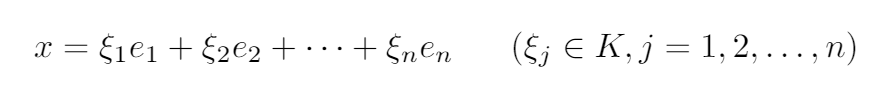# Linear spaces, the base of matrices calculationsThe basis for accelerating the big data models training. The last deep math posts are coming out, it’s a bit weird and difficult to read, but we need to know the basic rules of linear systems to be able to use them properly, so in this post, we explain some basic properties of linear spaces.

The last deep math posts are coming out, it’s a bit weird and difficult to read, but we need to know the basic rules of linear systems to be able to use them properly, so in this post, we explain some basic properties of linear spaces.

## Basis Components and Dimensions

By definition, a system of linearly independent vectors in a linear space _K _over a field K is called a basis for _K, _if given any x ϵ_ K _there exists a uniquely defined expansion:Expansion that should exist for every basis, self-generated.

For example, the identity matrix, created by n orthogonal vectors is a basis for the K_n _space.

When we are able to find a basis for our linear space, all the originally abstract operations become linear operations, which makes them easier to solve.

If in a linear space K, we can find n linearly independent vectors and no n+1 linearly independent vectors. Then n is called the dimension *of the space *K, which is called dimensional and the n vectors are the basis *of the space *K.

## Subspaces

Soppse that a set L of elements of a linear space Khas the following properties:

• If x ϵ Ly ϵ L, then x + y ϵ L.
• If x ϵ L *and *λ *is an element of the field *K, then λx ϵ L.

Then L is a set of elements with linear operations defined on it, this set is also a linear space and each L ϵ K *is called a *linear subspace of K.

If a basis is chosen in a subspace L, then we can always add additional vectors such as the system becomes a basis for all K.

## Basic Linear Algebra for Deep Learning and Machine Learning Python Tutorial

An introductory tutorial to linear algebra for machine learning (ML) and deep learning with sample code implementations in Python. Basic Linear Algebra for Deep Learning and Machine Learning Python Tutorial

## Mathematics for Machine Learning: Linear Algebra || Linear Algebra for Machine Learning

In this Mathematics for Machine Learning course you will learn everything you need to know about linear algebra for machine learning. First part of this linear algebra course you will find the basics of linear algebra and second part of this course discussed about advanced linear algebra. This will allow to understand machine learning from linear algebra hence mathematical point of view.

## Linear Algebra: The hidden engine of machine learning

The hidden engine of machine learning. Algebra is firstly taken from a book, written by Khwarizmi(780-850 CE), which is about calculation and equations.

## Most popular Data Science and Machine Learning courses — July 2020

Most popular Data Science and Machine Learning courses — August 2020. This list was last updated in August 2020 — and will be updated regularly so as to keep it relevant

## Linear Algebra for Machine Learning | Data Science | Machine Learning

Linear algebra is one of the most important topics in machine learning. In this article, I will introduce you to the basic concepts of linear algebra for machine learning using NumPy.